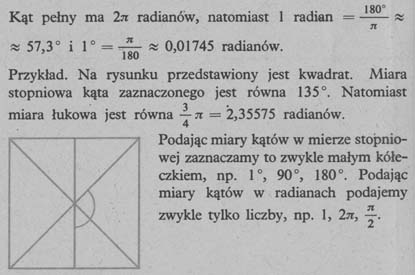# A step measure and an arc measure of an angle

Full angle has 360 degrees, a half angle 180 degrees. When the half angle divides into 180 equal angles, then we get the angle, which is a unit of incremental measure and is called degree.

The unit of measure for the arc angle is radian.

One radian is the angle defined by an arc equal in length to the radius.